Updating search results...

# 22 Results

View
Selected filters:
• CCSS.Math.Content.7.EE.B.4a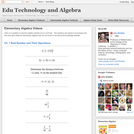Conditional Remix & Share Permitted
CC BY-NC
Rating
0.0 stars

Visually searchable database of Algebra 1 videos. Click on a problem and watch the solution on YouTube. Copy and paste this material into your CMS. Videos accompany the open Elementary Algebra textbook published by Flat World Knowledge.

Subject:
Algebra
Mathematics
Material Type:
Lecture
Lecture Notes
Provider:
Individual Authors
Provider Set:
Individual Authors
Author:
John Redden
04/29/2012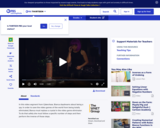Educational Use
Rating
0.0 stars

This video segment from Cyberchase introduces the idea of inverse operations as Bianca imagines herself as a spy.

Subject:
Mathematics
Material Type:
Lecture
Provider:
PBS LearningMedia
Provider Set:
PBS Learning Media: Multimedia Resources for the Classroom and Professional Development
Author:
U.S. Department of Education
WNET
07/10/2008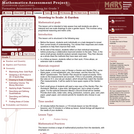Only Sharing Permitted
CC BY-NC-ND
Rating
0.0 stars

This lesson unit is intended to help assess how well students are able to interpret and use scale drawings to plan a garden layout. This involves using proportional reasoning and metric units.

Subject:
Algebra
Geometry
Mathematics
Ratios and Proportions
Material Type:
Assessment
Lesson Plan
Provider:
Shell Center for Mathematical Education
Provider Set:
Mathematics Assessment Project (MAP)
04/26/2013Unrestricted Use
CC BY
Rating
0.0 stars

This task is the second in a series of three tasks that use inequalities in the same context at increasing complexity in 6th grade, 7th grade and in HS algebra. Students write and solve inequalities, and represent the solutions graphically.

Subject:
Algebra
Mathematics
Material Type:
Activity/Lab
Provider:
Illustrative Mathematics
Provider Set:
Illustrative Mathematics
Author:
Illustrative Mathematics
05/01/2012Unrestricted Use
CC BY
Rating
0.0 stars

This problem asks the students to represent a sequence of operations using an expression and then to write and solve simple equations. The problem is posed as a game and allows the students to visualize mathematical operations. It would make sense to actually play a similar game in pairs first and then ask the students to record the operations to figure out each other's numbers.

Subject:
Algebra
Mathematics
Material Type:
Activity/Lab
Provider:
Illustrative Mathematics
Provider Set:
Illustrative Mathematics
Author:
Illustrative Mathematics
08/07/2012Conditional Remix & Share Permitted
CC BY-NC
Rating
0.0 stars

Four full-year digital course, built from the ground up and fully-aligned to the Common Core State Standards, for 7th grade Mathematics. Created using research-based approaches to teaching and learning, the Open Access Common Core Course for Mathematics&nbsp;is designed with student-centered learning in mind, including activities for students to develop valuable 21st century skills and academic mindset.

Subject:
Mathematics
Material Type:
Full Course
Provider:
Pearson
10/06/2016Conditional Remix & Share Permitted
CC BY-NC
Rating
0.0 stars

Algebraic Reasoning

Type of Unit: Concept

Prior Knowledge

Students should be able to:

Add, subtract, multiply, and divide rational numbers.
Evaluate expressions for a value of a variable.
Use the distributive property to generate equivalent expressions including combining like terms.
Understand solving an equation or inequality as a process of answering a question: which values from a specified set, if any, make the equation or inequality true?
Write and solve equations of the form x+p=q and px=q for cases in which p, q, and x are non-negative rational numbers.
Understand and graph solutions to inequalities x&lt;c or x&gt;c.
Use equations, tables, and graphs to represent the relationship between two variables.
Relate fractions, decimals, and percents.
Solve percent problems included those involving percent of increase or percent of decrease.

Lesson Flow

This unit covers all of the Common Core State Standards for Expressions and Equations in Grade 7. Students extend what they learned in Grade 6 about evaluating expressions and using properties to write equivalent expressions. They write, evaluate, and simplify expressions that now contain both positive and negative rational numbers. They write algebraic expressions for problem situations and discuss how different equivalent expressions can be used to represent different ways of solving the same problem. They make connections between various forms of rational numbers. Students apply what they learned in Grade 6 about solving equations such as x+2=6 or 3x=12 to solving equations such as 3x+6=12 and 3(x−2)=12. Students solve these equations using formal algebraic methods. The numbers in these equations can now be rational numbers. They use estimation and mental math to estimate solutions. They learn how solving linear inequalities differs from solving linear equations and then they solve and graph linear inequalities such as −3x+4&lt;12. Students use inequalities to solve real-world problems, solving the problem first by arithmetic and then by writing and solving an inequality. They see that the solution of the algebraic inequality may differ from the solution to the problem.

Subject:
Algebra
Mathematics
Material Type:
Unit of Study
Provider:
Pearson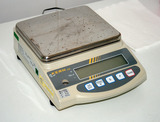Conditional Remix & Share Permitted
CC BY-NC
Rating
0.0 stars

Students use algebraic expressions and equations to represent rules of thumb involving measurement. They use properties of operations and the relationships between fractions, decimals, and percents to write equivalent expressions.Key ConceptsExpressions and equations are different. An expression is a number, a variable, or a combination of numbers and variables. Some examples of expressions are:74x5a + b3(2m + 1)In Grade 7, the focus is on linear expressions. A linear expression is a sum of terms that are either rational numbers or a rational number times a variable (with an exponent of either 0 or 1). If an expression contains a variable, it is called an algebraic expression. To evaluate an expression, each variable is replaced with a given value.Equivalent expressions are expressions for which a given value can be substituted for each variable and the value of the expressions are the same.An equation is a statement that two expressions are equal. An equation can be true or false. To solve an equation, students find the value of the variable that makes the equation true.Students solve an equation that involves finding 10% of a number. They see that finding 10% of the number is the same as finding 0.1 of the number, or finding 110 of the number.Goals and Learning ObjectivesWrite expressions and equations to represent real-world situations.Evaluate expressions for given values of a variable.Use properties of operations to write equivalent expressions.Solve one-step equations.Check the solution to an equation.

Subject:
Algebra
Material Type:
Lesson Plan
09/21/2015Conditional Remix & Share Permitted
CC BY-NC
Rating
0.0 stars

Students explore the effects of wind on a plane&#39;s time and distance and represent these situations using algebraic expressions and equations. They use terms with positive, negative, and zero coefficients.Key ConceptsIn this lesson, students show what they remember from Grade 6 about writing expressions and solving one-step equations. They use what they learned earlier in Grade 7 about adding and subtracting integers. They extend these concepts to write and interpret an expression with a negative coefficient.Goals and Learning ObjectivesReview addition and subtraction of integers.Review the relationship between distance, time, and speed.Write an algebraic expression for distance in terms of time, t.Write a term with a negative coefficient.Review solving a one-step equation using the multiplication property of equality.

Subject:
Algebra
Material Type:
Lesson Plan
09/21/2015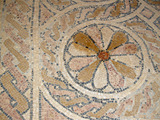Conditional Remix & Share Permitted
CC BY-NC
Rating
0.0 stars

Students write expressions for geometric situations. They examine how different equivalent expressions can show different ways of thinking about the same problem.Key ConceptsStudents use their previous knowledge of how to find the perimeter and area of squares and rectangles. They write algebraic expressions for the perimeter and area of geometric figures. They examine how equivalent expressions, used to represent a problem situation, give clues to the approach the writer of the expression used to solve the problem. In the Challenge Problem, they use the distributive property to find the solution.ELL: For ELLs, access prior knowledge by writing the words area and perimeter on the board. Have students create concept maps associated with area and perimeter. Record students&#39; responses on large poster paper that you can display in the room. The goal is to generate a list of words that students can use as a reference.Goals and Learning ObjectivesAccess prior knowledge of how to find the perimeter and area of squares and rectangles.Write algebraic expressions for finding perimeter or area of figures.Identify equivalent expressions.

Subject:
Geometry
Material Type:
Lesson Plan
09/21/2015Conditional Remix & Share Permitted
CC BY-NC
Rating
0.0 stars

Students use inequalities to solve real-world problems. They see that the solution of the algebraic inequality may differ from the solution to the problem it represents. For example, a fractional number or a negative number may not be an appropriate solution for a word problem.Students complete a Self Check. They are given an algebraic inequality that they need to solve. They then write and solve a word problem that the inequality could represent.Key ConceptsIn this lesson, students write and solve an algebraic inequality that matches a situation given in a word problem. They then interpret that algebraic solution in the context of the problem. For example, students write and solve an algebraic inequality to represent the number of T-shirts that can be bought given a certain amount of money and another purchase. The inequality produces the solution t &lt; 2.5. Since a fractional part of a T-shirt does not make sense, students reason that 2 is the greatest number of T-shirts that can be purchased.Goals and Learning ObjectivesInterpret the solution to an algebraic inequality within the context of a word problem.

Subject:
Algebra
Material Type:
Lesson Plan
09/21/2015Conditional Remix & Share Permitted
CC BY-NC
Rating
0.0 stars

Students work with a partner to revise their work on the Self Check. Students work with their partner to do activities that involve using expressions and equations to solve problems.Key ConceptsStudents will use what they have learned so far in this unit about writing expressions as well as writing and using equations to solve problems.Goals and Learning ObjectivesUse expressions and equations to solve problems.

Subject:
Algebra
Material Type:
Lesson Plan
09/21/2015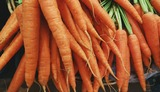Conditional Remix & Share Permitted
CC BY-NC
Rating
0.0 stars

Students solve real-world problems by writing and solving equations. Students estimate the solution and determine if the estimate is reasonable before finding the exact solution. They write the solution as a complete sentence.Students complete a Self Check.Key ConceptsStudents solve real-world problems by first estimating the solution and assessing the reasonableness of the solution. Next, they write an equation to solve the problem and then use the properties of equality to solve the equation. Students write the solution to the problem as a complete sentence.Goals and Learning ObjectivesWrite equations to solve multi-step real-life problems involving rational numbers.Solve equations using addition, subtraction, multiplication, and division of rational numbers.Use estimations strategies to estimate the solution and determine if the estimate is reasonable.Write the solution as a complete sentence.

Subject:
Algebra
Material Type:
Lesson Plan
09/21/2015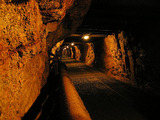Conditional Remix & Share Permitted
CC BY-NC
Rating
0.0 stars

Students extend what they learned about solving equations in Grade 6. They learn to solve equations that require them to use both the addition and the multiplication properties of equality. They use what they know about solving equations such as 2x = 6 and x + 3 = 7 to solve equations such as 2x + 3 = 8. They connect solving problems using arithmetic to solving problems using equations. They solve equations containing both positive and negative rational numbers.Key ConceptsAddition property of equality: If a = b, then a + c = b + c.Multiplication property of equality: If a = b, then ac = bc.For any equation, add or subtract the same value from both sides of the equation and the equation will still be true.For any equation, multiply or divide both sides of the equation by the same value and the equation will still be true.In this lesson, students use both properties to solve equations. They then solve equations that contain both positive and negative rational numbers.Goals and Learning ObjectivesSolve equations using both the addition and multiplication properties of equality.Relate solving problems using arithmetic to solving problems using equations.Solve equations containing both positive and negative rational numbers.

Subject:
Algebra
Material Type:
Lesson Plan
09/21/2015Conditional Remix & Share Permitted
CC BY-NC
Rating
0.0 stars

Putting Math to Work

Type of Unit: Problem Solving

Prior Knowledge

Students should be able to:

Solve problems involving all four operations with rational numbers.
Write ratios and rates.
Write and solve proportions.
Solve problems involving scale.
Write and solve equations to represent problem situations.
Create and interpret maps, graphs, and diagrams.
Use multiple representations (i.e., tables, graphs, and equations) to represent problem situations.
Calculate area and volume.
Solve problems involving linear measurement.

Lesson Flow

Students apply and integrate math concepts they have previously learned to solve mathematical and real-world problems using a variety of strategies. Students have opportunities to explore four real-world situations involving problem solving in a variety of contexts, complete a project of their choice, and work through a series of Gallery problems.

First, students utilize their spatial reasoning and visualization skills to find the least number of cubes needed to construct a structure when given the front and side views. Then, students select a project to complete as they work through this unit to refine their problem-solving skills. Students explore the relationship between flapping frequency, amplitude, and cruising speed to calculate the Strouhal number of a variety of flying and swimming animals. After that, students explore the volume of the Great Lakes, applying strategies for solving volume problems and analyzing diagrams. Next, students graphically represent a virtual journey through the locks of the Welland Canal, estimating the amount of drop through each lock and the distance traveled. Students have a day in class to work on their projects with their group.

Then, students have two days to explore Gallery problems of their choosing. Finally, students present their projects to the class.

Subject:
Mathematics
Material Type:
Unit of Study
Provider:
PearsonConditional Remix & Share Permitted
CC BY-NC
Rating
0.0 stars

Students explore the relationship between the flapping frequency, the amplitude, and the cruising speeds of a variety of animals to calculate their Strouhal numbers.Key ConceptsStudents are expected to use the mathematical skills they have acquired in previous lessons or in previous math courses. The lessons in this unit focus on developing and refining problem-solving skills. Students will:Try a variety of strategies to approaching different types of problems.Devise a problem-solving plan and implement their plan systematically.Become aware that problems can be solved in more than one way.See the value of approaching problems in a systematic manner.Communicate their approaches with precision and articulate why their strategies and solutions are reasonable.Make connections between previous learning and real-world problems.Create efficacy and confidence in solving challenging problems in a real-world setting.Goals and Learning ObjectivesAnalyze the relationship between the variables in an equation.Write formulas to show how variables relate.Communicate findings using multiple representations including tables, charts, graphs, and equations.

Subject:
Algebra
Material Type:
Lesson Plan
09/21/2015Conditional Remix & Share Permitted
CC BY-NC
Rating
0.0 stars

How much water is in the Great Lakes? Students read and interpret a diagram that shows physical features of the Great Lakes and answer questions based on the diagram. They find the volume of each of the Great Lakes, as well as all five lakes combined, and make a bar graph to represent the volumes.Key ConceptsStudents are expected to use the mathematical skills they have acquired in previous lessons or in previous math courses. The lessons in this unit focus on developing and refining problem-solving skills.Students will:Try a variety of strategies to approaching different types of problems.Devise a problem-solving plan and implement their plan systematically.Become aware that problems can be solved in more than one way.See the value of approaching problems in a systematic manner.Communicate their approaches with precision and articulate why their strategies and solutions are reasonable.Make connections between previous learning and real-world problems.Create efficacy and confidence in solving challenging problems in a real-world setting.Goals and Learning ObjectivesRead and interpret graphs and diagrams.Solve problems involving volume.

Subject:
Geometry
Material Type:
Lesson Plan
09/21/2015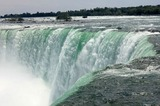Conditional Remix & Share Permitted
CC BY-NC
Rating
0.0 stars

Students first create a diagram that represents the distance a ship drops in each of a series of locks. Students create their diagrams based on a video of an actual ship traveling through the locks. Students need to use contextual clues in order to determine the relative drops in each of the locks.Key ConceptsStudents are expected to use the mathematical skills they have acquired in previous lessons or in previous math courses. The lessons in this unit focus on developing and refining problem-solving skills.Students will:Try a variety of strategies to approaching different types of problems.Devise a problem-solving plan and implement their plan systematically.Become aware that problems can be solved in more than one way.See the value of approaching problems in a systematic manner.Communicate their approaches with precision and articulate why their strategies and solutions are reasonable.Make connections between previous learning and real-world problems.Create efficacy and confidence in solving challenging problems in a real-world setting.Goals and Learning ObjectivesRead and interpret maps, graphs, and diagrams.Solve problems that involve linear measurement.Estimate length.Critique a diagram.

Subject:
Algebra
Material Type:
Lesson Plan
09/21/2015Conditional Remix & Share Permitted
CC BY-NC
Rating
0.0 stars

Students critique the diagrams of other students from the previous lesson and receive feedback about their own diagrams. Students revise their diagrams from the first part of the lesson based on the feedback they receive.Key ConceptsStudents are expected to use the mathematical skills they have acquired in previous lessons or in previous math courses. The lessons in this unit focus on developing and refining problem-solving skills. Students will:Try a variety of strategies to approaching different types of problems.Devise a problem-solving plan and implement their plan systematically.Become aware that problems can be solved in more than one way.See the value of approaching problems in a systematic manner.Communicate their approaches with precision and articulate why their strategies and solutions are reasonable.Make connections between previous learning and real-world problems.Create efficacy and confidence in solving challenging problems in a real-world setting.Goals and Learning ObjectivesRead and interpret maps, graphs, and diagrams.Solve problems that involve linear measurement.Estimate length.Critique a diagram.SWD: Some students with disabilities will benefit from a preview of the goals in each lesson. Students can highlight the critical features and/or concepts and will help them to pay close attention to salient information. Students need to know their goal is to develop and refine their problem solving skills.

Subject:
Mathematics
Material Type:
Lesson Plan
09/21/2015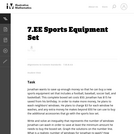Unrestricted Use
CC BY
Rating
0.0 stars

The purpose of this task is to present students with a context that can naturally be represented with an inequality and to explore the relationship between the context and the mathematical representation of that context; thus, this is an intended as an instructional task.

Subject:
Mathematics
Material Type:
Activity/Lab
Provider:
Illustrative Mathematics
Provider Set:
Illustrative Mathematics
Author:
Illustrative Mathematics